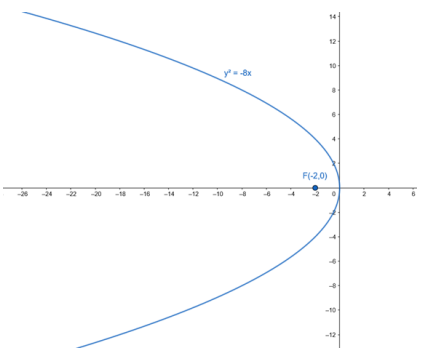# Find the equation of the parabola with vertex at the origin and focus at`
Question:

Find the equation of the parabola with vertex at the origin and focus at F(-2, 0).

Solution:

Vertex : A (0,0)

Given focus $F(-2,0)$ is of the form $F(-a, 0)$

For Vertex $A(0,0)$ and Focus $F(-a, 0)$, equation of parabola is

$y^{2}=-4 a x$

Here, a = 2

Therefore, equation of parabola,

$y^{2}=-8 x$